# Active and Reactive Powers Control of DFIG Based WECS Using PI Controller and Artificial Neural Network Based Controller

Active and Reactive Powers Control of DFIG Based WECS Using PI Controller and Artificial Neural Network Based Controller
Electrical Engineering Laboratory (LGE), University of M’sila, M’sila 28000, Algeria

Corresponding Author Email:
bilal.boudjellal@univ-msila.dz
Page:
31-38
|
DOI:
https://doi.org/10.18280/mmc_a.931-405
27 March 2019
|
Accepted:
10 October 2020
|
Published:
31 December 2020
| Citation

OPEN ACCESS

Abstract:

The purpose of this study is to improve the control performance of a Doubly Fed Induction Generator (DFIG) in a Wind Energy Conversion System (WECS) by using both of the conventional Proportional-Integral (PI) controllers and an Artificial Neural Network (ANN) based controllers. The rotor-side converter (RSC) voltages are controlled using a stator flux oriented control (FOC) to achieve an independent control of the active and reactive powers, exchanged between the stator of the DFIG and the power grid. Afterward, the PI controllers of the FOC are replaced with two ANN based controllers. A Maximum Power Point Tracking (MPPT) control strategy is necessary in order to extract the maximum power from the of wind energy system. A simulation model was carried out in MATLAB environment under different scenarios. The obtained results demonstrate the efficiency of the proposed ANN control strategy.

Keywords:

active and reactive powers control, Artificial Neural Network controller, wind turbine, field oriented control, doubly-fed induction generator (DFIG), Proportional-Integral (PI) controller, wind energy conversion system (WECS)

1. Introduction

Variable speed wind turbines are generally a megawatt class, especially in wind farms. Currently, doubly fed induction generator based wind turbines are the preferred choice for high capacity wind farms due of its unique features such as flexible control, low cost, high efficiency and ability to independently control active and reactive powers exchange with the grid system [1-3]. The DFIG stator winding is connected directly to the grid, while its rotor is connected indirectly to the same grid via a back-to-back power converter. The back-to-back converter has to handle only a fraction (30%) of the total power .

Regardless of the large advantages of DFIG, there is a challenging control problem, because of its nonlinear multi-variable system and being highly coupled. Therefore, a decoupled active and reactive powers control technique is mandatory to achieve a constant operating frequency at variable wind speeds [4, 5].

Recently, several papers have studied the control techniques of DFIG [6-14]. Some used a simple field-oriented vector control using PI controllers [6-10]. Others used nonlinear model predictive control . In addition, others used intelligent control techniques, such as genetic algorithm, fuzzy logic and artificial neural network [12-14].

This paper proposes a new control of a DFIG in a WECS using an ANN based controller. This new control is compared with the conventional PI controller. A brief overview of the artificial neural network is presented in section 2, followed by a brief description of the system including its modeling in sections 3. Both of the PI based control technique and ANN based control technique are presented in sections 4. Obtained simulation results of the proposed control techniques are presented and discussed in section 5.

2. An Artificial Neural Networks Overview

Artificial Neural Networks (ANNs) are mathematical representations inspired by the functioning of the human brain. Thus, ANNs are capable of modeling very complex functions . ANNs have been applied successfully in various fields of modeling and prediction in various implications, also are being implemented to an increasing number of real-world issues of high complexity in many science and engineering applications. The ANN takes a number of artificial neurons, connected in densely parallel or in sequence arrangement in order to recognize the highly complex patterns occurring within the available data. Basic component of a neural network is the artificial neuron, also called node, which is a model of a biological neuron. Those nodes are combined to form a layer within the ANN.

There are several types of ANNs, the most commonly implemented type of ANNs is Feed-Forward Neural Networks (FFNN), which consist of an input layer, one or more hidden layers, and an output layer. Each one of the layers consists of several neurons (Figure 1). Each neuron processes its input and generates an output value, which transmitted to the neurons in the subsequent layer. All neurons and layers are arranged in a feed-forward manner, and no feedback connections are allowed. The number of the hidden layers and the number of the neurons in each hidden layer depend mainly on the complexity of the issue. Figuring out the optimum number of neurons in hidden layer for ANN model is important task to avoid under-fitting and over-fitting [14-17].

There are many training algorithms available. One of the most popular and successful training algorithms for FFNNs is called back propagation. Herein, the Levenberg-Marquardt backpropagation training algorithm was chosen due to the fact that it is the fastest backpropagation algorithm in the toolbox, and is highly recommended as a first-choice supervised algorithm [18-20].

## 1.pngFigure 1. Schematic representation of a multilayer Neural Network

3. Wind Energy Conversion System Overview

The studied system is shown in Figure 2. The system components are: the wind turbine, induction generator, voltage source converter and the electrical grid. The process of WECS is to transforms wind energy into mechanical energy by wind turbine blades and, eventually, into electrical energy through the generator.

Wind turbines use a doubly fed induction generator consisting of a wound rotor induction generator and an AC/DC/AC converter. The stator winding is connected directly to the grid while the rotor is fed at variable frequency, phase, and magnitude through the converter.

## 2.pngFigure 2. Wind energy conversion system based on a DFIG

3.1 Modeling of wind turbine

The mathematical equation of the mechanical power extracted from the wind power can expressed as follows:

${{P}_{t}}=\frac{1}{2}\rho {{R}^{2}}{{v}^{3}}{{C}_{p}}\left( \lambda ,\beta \right)$        (1)

The mechanical torque Tt is:

${{T}_{t}}=\frac{{{P}_{t}}}{{{\Omega }_{t}}}=\frac{1}{2\lambda }\rho \pi {{R}^{3}}{{v}^{2}}{{C}_{p}}\left( \lambda ,\beta \right)$                          (2)

where, v is wind speed, ρ is air density (1,225 kg/m3), R is blade radius, t is angular speed of the turbine and Cp(λ,β) is the turbine power coefficient which is function of the pitch angle of the rotor blades β and the tip speed ratio λ.

The turbine power coefficient can be described as :

${{C}_{p}}\left( \lambda ,\beta \right)=\left( 0.35\text{ }-\text{ }0.0167\cdot \left( \beta -2 \right) \right)\cdot \sin \left( \frac{\pi \cdot \left( \lambda +0.1 \right)}{14.34-0.3\left( \beta -2 \right)} \right)-\left( 0.00184\cdot \left( \lambda -3 \right)\cdot \left( \beta -2 \right) \right)$             (3)

The maximum value of power coefficient Cp (Cp_max=0.35) is achieved for β equal to 2 degree and for λ equal to 7.07. Tip speed ratio λ is defined as:

$\lambda =\frac{\left( {{\Omega }_{t}}\cdot R \right)}{v}$         (4)

The system is composed by three-bladed wind turbine coupled to the rotor of the DFIG though a mechanical gearbox whose gear ratio G is chosen in order to set the generator shaft speed mec within a desired speed range.

The system dynamics is given by:

$J\frac{d{{\Omega }_{mec}}}{dt}={{T}_{g}}-{{T}_{em}}-f{{\Omega }_{mec}}$  (5)

where, Tg is the mechanical torque of the generator, J and f are the moment of inertia of the system and the friction coefficient.

The turbine model in the form of diagram blocks is represents in Figure 3.

## 3.pngFigure 3. Wind Turbine model

3.2 Modeling of double fed induction generator

Park mathematical model of the DFIG is given by the following Eqns. [2-8]:

\left\{ \begin{align} & {{v}_{sd}}=-{{R}_{s}}{{i}_{sd}}+\frac{d}{dt}{{\phi }_{sd}}-{{\omega }_{s}}{{\phi }_{sq}} \\ & {{v}_{sq}}=-{{R}_{s}}{{i}_{sq}}+\frac{d}{dt}{{\phi }_{sq}}+{{\omega }_{s}}{{\phi }_{sd}} \\ & {{v}_{rd}}={{R}_{r}}{{i}_{rd}}+\frac{d}{dt}{{\phi }_{rd}}-{{\omega }_{r}}{{\phi }_{rq}} \\ & {{v}_{rq}}={{R}_{r}}{{i}_{rq}}+\frac{d}{dt}{{\phi }_{rq}}+{{\omega }_{r}}{{\phi }_{rd}} \\ \end{align} \right.             (6)

\left\{ \begin{align} & {{\phi }_{sd}}=-{{L}_{s}}{{i}_{sd}}+M{{i}_{rd}} \\ & {{\phi }_{sq}}=-{{L}_{s}}{{i}_{sq}}+M{{i}_{rq}} \\ & {{\phi }_{rd}}={{L}_{r}}{{i}_{rd}}-M{{i}_{sd}} \\ & {{\phi }_{rq}}={{L}_{r}}{{i}_{rq}}-M{{i}_{sq}} \\ \end{align} \right.           (7)

The DFIG electromagnetic torque can be expressed by:

${{T}_{em}}=\frac{pM}{{{L}_{s}}}\left( {{i}_{rd}}{{\phi }_{sq}}-{{i}_{rq}}{{\phi }_{sd}} \right)$       (8)

The stator active and reactive powers equations can be written as:

\left\{ \begin{align} & {{P}_{s}}=-{{v}_{sd}}{{i}_{sd}}-{{v}_{sq}}{{i}_{sq}} \\ & {{Q}_{s}}=-{{v}_{sq}}{{i}_{sd}}+{{v}_{sd}}{{i}_{sq}} \\ \end{align} \right.            (9)

here:

• vsd,vsq,vrd,vrq: stator and rotor voltage components.

• isd,isq,ird,irq: stator and rotor current components.

• Φsd, Φsq, Φrd, Φrq: stator and rotor flux components.

• ωsr: stator and rotor pulsations.

• Rs,Rr: are stator and rotor resistances.

• Ls,Lr are stator and rotor inductances.

• M is mutual inductance.

The stator active and reactive powers vector uncoupled control can be accomplished by orienting the Park frame (d,q) so that the (d) axis is aligned with the stator flux [2-8]. Hence, the stator flux becomes:

\left\{ \begin{align} & {{\phi }_{sd}}={{\phi }_{s}}=-{{L}_{s}}{{i}_{sd}}+M{{i}_{rd}} \\ & {{\phi }_{sq}}=0=-{{L}_{s}}{{i}_{sq}}+M{{i}_{rq}} \\ \end{align} \right.           (10)

By neglecting the stator windings resistances (for high power generators) the stator voltages equations become:

\left\{ \begin{align} & {{v}_{sd=0}} \\ & {{v}_{sq}}={{v}_{s}}={{\omega }_{s}}{{\phi }_{s}} \\ \end{align} \right.                  (11)

The stator currents depend on rotor currents via the following equation:

$\left\{ \begin{smallmatrix} {{i}_{sd}}=\frac{M}{{{L}_{s}}}{{i}_{rd}}-\frac{{{\phi }_{s}}}{{{L}_{s}}} \\ {{i}_{sq}}=\frac{M}{{{L}_{s}}}{{i}_{rq}} \end{smallmatrix} \right.$             (12)

The stator active and reactive powers can be written as:

$\left\{ \begin{smallmatrix} {{P}_{s}}=-{{v}_{s}}{{i}_{sq}}=-\frac{{{v}_{s}}M}{{{L}_{s}}}{{i}_{rq}} \\ {{Q}_{s}}=-{{v}_{s}}{{i}_{sd}}=-\frac{{{v}_{s}}M}{{{L}_{s}}}{{i}_{rd}}+\frac{{{v}_{s}}^{2}}{{{L}_{s}}{{\omega }_{s}}} \end{smallmatrix} \right.$          (13)

Combining (7) and (12) yields:

\left\{ \begin{align} & {{\phi }_{rd}}=\left( {{L}_{r}}-\frac{{{M}^{2}}}{{{L}_{s}}} \right){{i}_{rd}}+\frac{M}{{{L}_{s}}}{{\phi }_{s}}=\sigma {{L}_{r}}{{i}_{rq}}+\frac{M}{{{L}_{s}}}\phi \\ & {{\phi }_{rq}}=\left( {{L}_{r}}-\frac{{{M}^{2}}}{{{L}_{s}}} \right){{i}_{rq}}=\sigma {{L}_{r}}{{i}_{rq}} \\ \end{align} \right.              (14)

By substituting (14) in (6), we obtain:

$\left\{ \begin{smallmatrix} {{v}_{rd}}={{R}_{r}}{{i}_{rd}}+\sigma {{L}_{r}}\frac{d{{i}_{rd}}}{dt}-\sigma {{L}_{r}}{{\omega }_{r}}{{i}_{rq}} \\ {{v}_{rq}}={{R}_{r}}{{i}_{rq}}+\sigma {{L}_{r}}\frac{d{{i}_{rq}}}{dt}+\sigma {{L}_{r}}{{\omega }_{r}}{{i}_{rd}}+\frac{M}{{{L}_{s}}}{{\omega }_{r}}{{\phi }_{s}} \end{smallmatrix} \right.$              (15)

where, $\sigma =1-\frac{{{M}^{2}}}{{{L}_{s}}{{L}_{r}}}$ is leakage factor.

In steady state, the voltage expressions are given by:

$\left\{ \begin{smallmatrix} {{v}_{rd}}={{R}_{r}}{{i}_{rd}}+{{e}_{rd}} \\ {{v}_{rq}}={{R}_{r}}{{i}_{rq}}+{{e}_{rq}}+{{e}_{\phi }} \end{smallmatrix} \right.$                   (16)

$\left\{ \begin{matrix} {{e}_{rd}}=-\sigma {{L}_{r}}{{\omega }_{r}}{{i}_{rq}} \\ {{e}_{rq}}=\sigma {{L}_{r}}{{\omega }_{r}}{{i}_{rd}} \\ {{e}_{_{\phi }}}={}^{M}/{}_{Ls}{{\omega }_{r}}{{\phi }_{s}} \\\end{matrix} \right.$             (17)

These previous equations can represent the DFIG simplified Model scheme as shown in Figure 4.

## 4.pngFigure 4. DFIG Simplified Model scheme

4. Control Techniques

4.1 MPPT control strategy

The Maximum Power Point Tracking (MPPT) control strategy is mandatory in order to extract the maximum power from the available wind energy. The MPPT control strategy is based on adjusting the rotor speed to track the optimal reference power. The rotor speed can be adjusted by controlling electromagnetic torque of the DFIG rotor [3-9].

Figure 5 shows the model scheme of the MPPT control strategy.

## 5.pngFigure 5. Model scheme of MPPT control strategy

4.2 DFIG active and reactive powers control using PI based controllers

As shown in Figure 4, a field-oriented control can be applied to the DFIG to control the active and reactive powers independently using the rotor voltages.

Figure 6 shows the model scheme of the field oriented control of DFIG.

## 6.pngFigure 6. Model scheme of DFIG active and reactive powers control using PI based controllers

4.3 DFIG active and reactive powers control using artificial neural network based controllers

The main idea of this control is to use two Artificial Neural Networks based controllers to control stator active and reactive powers independently. Figure 7 shows the model scheme of DFIG active and reactive powers control using ANN based controllers. The two Artificial Neural Networks are a Multilayer Perceptron networks (MLP) with a structure of (2-7-1). The first ANN inputs are the measured and reference active power, and the output is the Park quadratic voltage v’rq. Inputs of the second ANN are the measured and reference reactive power, and the output is the Park direct voltage v’rd. The active and reactive powers are independently controlled. Stator active power (Ps) is controlled via rotor Park quadratic voltage (vrq), while stator reactive power (Qs) is controlled via rotor Park direct voltage (vrd).

The activation functions are the tan-sigmoid for input layer, the log-sigmoid for hidden layer and the linear for output layer.

## 7.pngFigure 7. Model scheme of DFIG active and reactive powers control using ANN based controllers

The training data are obtained with the help of a PI controller data and a simple stochastic search for optimal different references of the active and reactive powers and for different wind speed. In the end, we have acquired over than 12483 samples for as ANN training data.

5. Results and Discussion

The system modeling was developed and simulated in MATLAB/SIMULINK environment using the Simscape Power Systems toolbox for verifying the validity of the proposed control strategy. System parameters are given in Table 1.

Table 1. Simulation parameters

 Variable Value U 690 V (50 Hz) Vdc 1,200 V Lfs 121 µH Lfr 57.3 µH Lm 12.12 mH P 2 Rs 2.97 mΩ Rr 3.82 mΩ J 114 kg.m2

## 8a.png(a) active power control ANN

## 8b.png(b) reactive power control ANN

Figure 8. Training performance for DFIG

## 9.png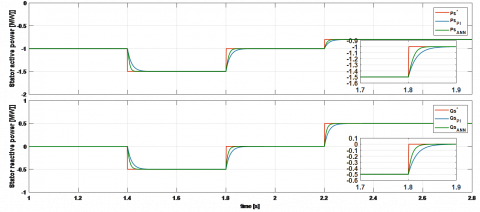Figure 9. DFIG stator active and reactive powers

## 10.png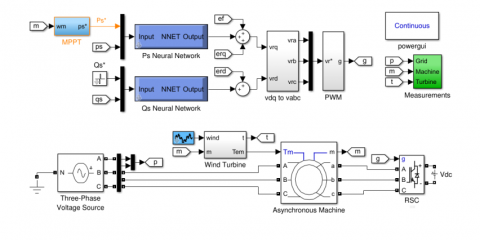Figure 10. WECS Simulink model block diagram

Figure 8 shows the training performance plot for DFIG active and reactive powers control ANNs. Training performance is based on mean square error between desired output and actual output of ANNs. From Figure 8a, it can be seen that best training performance for active power control ANN is equal to 0.30145e-5. Likewise, from Figure 8b, it can be seen that best training performance for reactive power control ANN is equal to 0.53278e-5.

Table 2. Comparative results between PI based control and ANN based control

 Variable PI ANN Active power response time [s] 0.071 0.028 Reactive power response time [s] 0.071 0.021

Figure 9 shows the step response simulation results of DFIG active and reactive powers control using the average model (IGBT’s of the RSC are represented by equivalent voltage sources). To verify the proposed control strategy, the ANN based control is compared with the PI based control. As can be seen below (Figure 9), stator active and reactive powers, tracks there references for both ANN based control and PI based control. However, the ANN based control provide a fast response and robust control. The overall measurement results are summarized in Table 2.

Figure 10 shows the detailed WECS Simulink model in MATLAB/Simulink environment. This model includes detailed representation of power electronic IGBT converters, in order to achieve more accuracy. Pulse width modulation frequency is set to 10 kHz.

A wind speed of 13 m/s has been applied on blades of the turbine, which corresponds to the nominal operation of the DFIG. The slip equal to -30% in hyper-synchronous mode, with a rotational speed equal to 1950 rpm, as shown in Figure 11.

As can be seen from Figure 12, both of the stator active power (Ps) and stator reactive power (Qs) are well regulated near their reference values.

The reference stator active power (Ps*) is generated using the MPPT control strategy. The WECS operates at unity power factor before the time t = 4.6 s and after the time t = 5.4 s because the reference reactive power Qs* is equal to 0 VAR. In the time interval of t = [4.6 s, 5.0 s] and t = [5.0 s, 5.4 s], the reference stator reactive power is set to −1 MVAR and 1 MVAR respectively. A little variation of stator active power (Ps*) can be observed, due to the important variation of rotor reactive power.

Figure 13 shows the temporal evolutions of different electrical powers of the WECS. It can be seen that, the stator reactive power Qs changes correspondently to the changing in the rotor current ird and rotor reactive power Qr. It is also noticed that when the stator reactive power Qs equal to 0 VAR (before the time t = 4.6 s and after the time t = 5.4 s), the rotor reactive power Qr is not equal to 0 VAR, because the DFIG requires a reactive power for its magnetization. It also can be observed that, the WECS operated in nominal conditions and generate a total power Pdfig equal to 3 MW, which demonstrate the performance of the proposed control strategy.

Figure 14 show stator and rotor voltage-current. It can be observed from Figure 14a, that the stator voltage-current are symmetric to ensure a unity power factor at the connection point of the stator with the electrical grid.

## 11.pngFigure 11. Generator rotational speed

## 12.png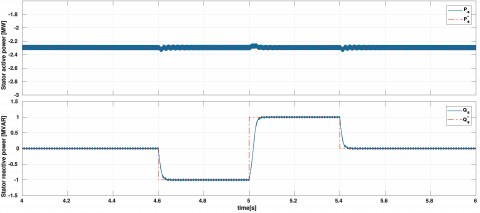Figure 12. Stator active and reactive powers

## 13.pngFigure 13. dq rotor currents, rotor active and reactive powers and total active and reactive powers

## 14a.png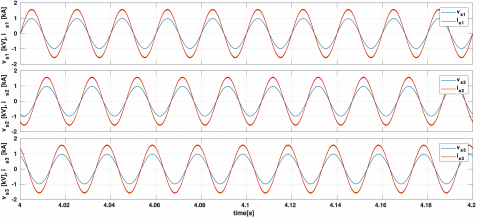(a) stator voltage-current

## 14b.png(b) rotor voltage-current

Figure 14. Voltage-current

## 15.png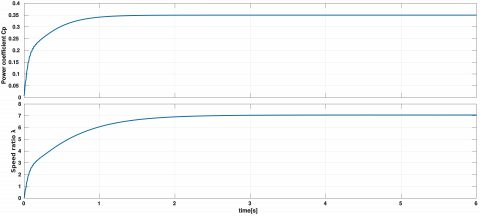Figure 15. Power coefficient Cp and speed ratio λ

Figure 15 shows respectively power coefficient Cp versus time and speed ratio λ versus time. As remarked, power coefficient Cp pursues its maximal values offering maximal mechanical power to the DFIG rotor. In addition, speed ratio λ is at its optimal value equal to 7.07 at β equal to.

6. Conclusion

In this paper, we examine the control performance improvement of a DFIG in a WECS using a new Artificial Neural Network controller based approach. A particular attention is paid to control the power flow exchanged between the DFIG stator and the power grid. A flux-oriented control is applied to the rotor side converter to achieve an independent control of the active and reactive powers. In order to extract the maximum power from the WECS a MPPT control strategy is used to generate the optimal reference power. To verify this new proposed control approach, the ANN based control is compared with a PI control. Simulations are performed in MATLAB/SIMULINK environment using a 3MW WECS. Based on the obtained results, it can be concluded that the proposed ANN based control strategy has successfully improved the control performance of the studied WECS system. Further study using hybrid ANN control strategy would be of interest to enhance the control strategy.

References

 Muller, S., Deicke, M., De Doncker, R.W. (2002). Doubly fed induction generator systems for wind turbines. IEEE Ind. Appl. Mag, 8(3): 26-33. http://dx.doi.org/10.1109/2943.999610

 Lei, Y.Z., Mullane, A., Lightbody, G., Yacamini, R. (2006). Modeling of the wind turbine with a doubly fed induction generator for grid integration studies. IEEE Trans. Energy Conversion, 21(1): 257-264. http://dx.doi.org/10.1109/TEC.2005.847958

 Gayathri, K., Jeevananthan, S. (2018). Candid inquest on modeling methods of doubly fed induction generator, and corroboration through simulation study. Modelling, Measurement and Control A, 91(4): 222-232. http://doi.org/10.18280/mmc_a.910408

 Lather, J.S., Dhillon, S.S., Marwaha, S. (2013). Modern control aspects in doubly fed induction generator based power systems: A review. International Journal of Advanced Research in Electrical, Electronics and Instrumentation Engineering, 2(6): 2149-2161.

 Hu. J.B., Heng, N., Xu, H.L., He, Y.K. (2011). Dynamic modeling and improved control of DFIG under distorted grid voltage conditions. IEEE Trans Energy Conversion, 26(1): 163-175. http://doi.org/10.1109/TEC.2010.2071875

 Poitiers, F., Machmoum, M., Doeuff, R.L., Zaim, M.E. (2001). Control of a doubly-fed induction generator for wind energy conversion systems. International Journal of Renewable Energy Engineering, pp. 373-378.

 Boulâam, K., Boukhelifa, A. (2013). Output power control of a wind energy conversion system based on a doubly fed induction generator. International Renewable and Sustainable Energy Conference (IRSEC), Ouarzazate, pp. 292–297. http://doi.org/10.1109/IRSEC.2013.6529707

 Boudjellal, B., Ferahtia, S. (2013). Field oriented control of active and reactive power of a DFIG with MPPT for variable speed wind energy conversion. International Conference on Power Electronics and their Applications.

 Farid, B., Rachide, A., Lokmane, B.M. (2014). Control of the doubly Fed Induction Generator in WECS. Power Engineering and Renewable Energy (ICPERE), Bali, pp. 25-30. http://doi.org/10.1109/ICPERE.2014.7067229

 Mishra, R., Saha, T.K. (2018). Operation in distributed power generation scheme with transition of control between stand-alone and grid connected modes. Modelling, Measurement and Control A, 91(2): 48-53. http://doi.org/10.18280/mmc_a.910203

 Ouari, K., Rekioua, T., Ouhrouche, M. (2013). Nonlinear Model Predictive Controller of a variable speed wind turbine driven Doubly Fed Induction Generator. J. Electrical Systems, 9(2): 243-255.

 Priyadarshini, J., Karthika, J. (2014). Neural network based MPPT for high performance wind generator using DFIG. Information Communication and Embedded Systems (ICICES), Chennai, pp. 1-5. http://doi.org/10.1109/ICICES.2014.7034187

 Chaiba, A. (2013). A neuro-fuzzy control based torque tracking approach for doubly fed induction generator. Power Engineering Energy and Electrical Drives (POWERENG), Istanbul, pp. 192-197. http://doi.org/10.1109/PowerEng.2013.6635605

 Kasiri, H., Abadeh, M.S., Momeni, H.R. (2012). Optimal estimation and control of WECS via a Genetic Neuro Fuzzy Approach. Energy, 40(1): 438-444. http://doi.org/10.1016/j.energy.2012.01.022

 Machavarapu, S., Mannam, V.G.R., Pulipaka, V.R.R. (2018). Improvement of multi-machine power system stability using artificial intelligent power system stabilizer. Modelling, Measurement and Control A, 91(3): 145-151. http://doi.org/10.18280/mmc_a.910307

 Badrnezhad, R., Mirzab, B. (2014). Modeling and optimization of cross-flow ultrafiltration using hybrid neural network-genetic algorithm approach. Journal of Industrial and Engineering Chemistry, 20(2): 528-543. http://doi.org/10.1016/j.jiec.2013.05.012

 Wang, L. (2005). A hybrid genetic algorithm–neural network strategy for simulation optimization. Applied Mathematics and Computation, 170(2): 1329-1343. http://doi.org/10.1016/j.amc.2005.01.024

 Chen, F.C. (1990). Back-propagation neural networks for nonlinear self-tuning adaptive control. IEEE, Control Systems Magazine, 10(3): 44-48. http://doi.org/10.1109/37.55123

 Thapa, B.K., Jones, B., Zhu, Q.M. (2000). Non-linear control with neural networks. Fourth International Conference on Knowledge-Based Intelligent Engineering Systems and Allied Technologies. Proceedings (Cat. No.00TH8516), Brighton, UK, 2(4): 868-873. http://doi.org/10.1109/KES.2000.884184

 Lourakis, I.M.A. (2005). A Brief description of the Levenberg-Marquardt algorithm implemented by levmar. Institute of Computer Science Foundation for Research and Technology, Greece.